# C++ Program to Find the Area and Perimeter of a Square

In this article, you will learn and get code for finding the area and perimeter of a square in C++ programming. Here is the list of approaches used to create the program:

Before starting the program, let's first see the formula to calculate the area and perimeter of a square.

### Area of a Square Formula

To find the area of a square, use the following formula:

`area = len*len`

len indicates the length of the square. Because all the sides of a square are the same length, take the length of each side and square it.That will be the area.

### Square Perimeter Formula

Use the following formula to calculate the perimeter of a square:

`perimeter = 4*len`

len indicates length. Now let's move on to the program.

## In C++, calculate the area of a square

To calculate the area of a square in C++ programming, you have to ask the user to enter the side length of the square. With this side length, apply the formula as given above and initialize its value to a variable say area and print its value as output as shown here in the following program.

```#include<iostream>
using namespace std;
int main()
{
float len, area;
cout<<"Enter the Length of Square: ";
cin>>len;
area = len*len;
cout<<"\nArea = "<<area;
cout<<endl;
return 0;
}```

This program was built and runs under the Code::Blocks IDE. Here is the initial snapshot of the sample run: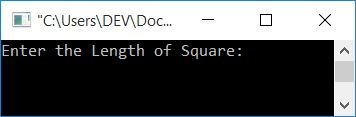Now enter the value of the length of a square, say 5, to find its area as shown in the final snapshot of the sample run given below: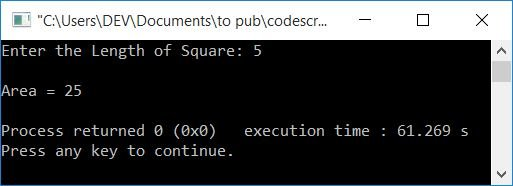### Find the Area of a Square in C++ Using a Function

This program does the same job as the previous one. The only difference is that this program finds the area of a square using a user-defined function named areaOfSquare(). It takes the length of the square as its argument and returns the area value of the square based on the length value passed as its argument.

```#include<iostream>
using namespace std;
float areaOfSquare(float);
int main()
{
float len, area;
cout<<"Enter the Length of Square: ";
cin>>len;
area = areaOfSquare(len);
cout<<"\nArea = "<<area;
cout<<endl;
return 0;
}
float areaOfSquare(float len)
{
return (len*len);
}```

This program will produce the same output as the previous one.

## In C++, find the perimeter of a square

Now let's create a program to find the perimeter of a square without using any functions.

```#include<iostream>
using namespace std;
int main()
{
float len, per;
cout<<"Enter the Length of Square: ";
cin>>len;
per = 4*len;
cout<<"\nPerimeter = "<<per;
cout<<endl;
return 0;
}```

Here is its sample run: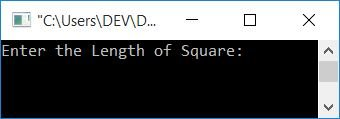Supply the length of any side of the square to find and print its perimeter, as shown in the following snapshot: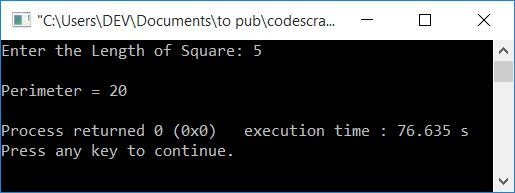### Find the perimeter of a square in C++ using a function

This program also does the same job as the previous one. It uses a function named perOfSquare() that calculates the perimeter of a square based on the length passed as its argument.

```#include<iostream>
using namespace std;
float perOfSquare(float);
int main()
{
float len;
cout<<"Enter the Length of Square: ";
cin>>len;
cout<<"\nPerimeter = "<<perOfSquare(len);
cout<<endl;
return 0;
}
float perOfSquare(float len)
{
return (4*len);
}```

## In C++, find the area and perimeter of a square using the class

This program finds both the area and perimeter of a square using a class and an object.

```#include<iostream>
using namespace std;
class CodesCracker
{
private:
float len;
public:
void getData();
float findArea();
float findPerimeter();
};
void CodesCracker::getData()
{
cout<<"Enter the Length of Square: ";
cin>>len;
}
float CodesCracker::findArea()
{
return (len*len);
}
float CodesCracker::findPerimeter()
{
return (4*len);
}
int main()
{
CodesCracker c;
float area, per;
c.getData();
area = c.findArea();
per = c.findPerimeter();
cout<<"\nArea = "<<area;
cout<<"\nPerimeter = "<<per;
cout<<endl;
return 0;
}```

Here's an example run with user input of 5: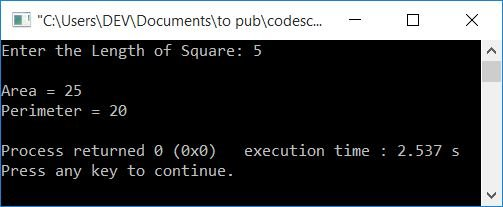#### The same program in different languages

C++ Quiz

« Previous Program Next Program »